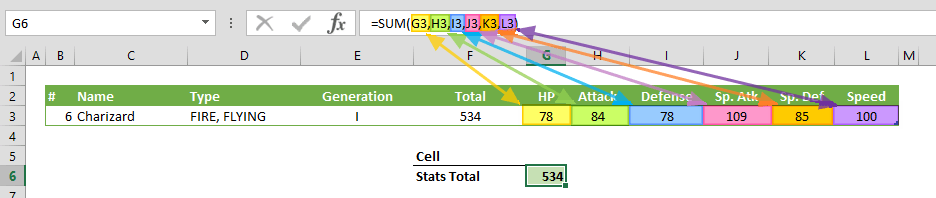The SUM function can sum up the values in a range of cells. In this guide, we’re going to show you how to use the SUM function and also throw in some tips and error handling methods.

• All versions

# Syntax

SUM(number1,[number2],...)

# Arguments

 number1 The first number or numbers you want to add. You can put a static number like 4, a cell reference like B6, or a cell range like B2:B8. [number2] Optional. The second number or numbers you want to add up to 255 numbers.

Examples

## Example 1

=SUM(78,84,78,109,85,100)
formula simply sums all numbers in the arguments.## Example 2

=SUM(G3,H3,I3,J3,K3,L3)
formula sums each value in referred cells.## Example 3

=SUM(G3:L3)
formula sums values in each cells in referred range. Any valid range can be used with the SUM function.# Tips

• The SUM function ignores the string values. However, please keep in mind that date or time values are serial numbers for Excel, not strings.
• Excel's AutoSum feature automatically adds the SUM formula by default. To apply the SUM function automatically:
• Select the range you want to sum as well as empty cell or cells for the SUM
• Press Alt + = (equal sign while pressing Alt key) combination
• To calculate the running totals use the SUM function with a mixed reference: How to calculate running totals in Excel Tables

# Issues

## #####

The column is too narrow for the result of the function. The solution is to increase the width of the column.

## Error

If there is an error in referred cells or ranges, the SUM function returns the same error.